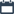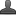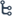## Thursday, October 8, 2020

•October 08, 2020
••, , , , ,
•No comments

## Key Points of Knowing Our Numbers

Hi friends and my dear students! In this post, I have covered Knowing Our Numbers Key Points Class -6,Notes,concept. After Reading Key Points Knowing Our Numbers, Please do share it with your friends. You can Learn Maths for All Classes here.Knowing Our Numbers Key Points Class - 6

## Knowing Our Numbers Key Points Class - 6

* Given two numbers, one with more digits is the greater number. If the number of digits in two given numbers is the same, that number is larger, which has a greater leftmost digit.

* Informing numbers from given digits, we should be careful to see if the conditions under which the numbers are to be formed are satisfied. Thus, to form the greatest four-digit number from 7, 8, 3, 5 without repeating a single digit, we need to use all four digits, the greatest number can have only 8 as the leftmost digit.

* The smallest four-digit number is 1000 (one thousand), It follows the largest three-digit number 999. Similarly, the smallest five-digit number is 10,000. It is ten thousand and follows the largest four-digit number 9999. Further, the smallest six-digit number is 1,00,000. It is one Lakh and follows the largest five-digit number 99,999. This carries on for higher digit numbers in a similar manner.

Trigonometry  Introduction

Trignometry Exercise - 11.1 Solutions

Trignometry Exercise - 11.1 Solutions Part - 1

* Use of commas helps in reading and writing large numbers. In the Indian system of numeration, we have commas after 3 digits starting from the right and thereafter every 2 digits. The commas after 3rd, 5th, and digits to separate thousand, lake, and crore respectively. In the International system of numeration, commas are placed after every 3 digits starting from the right. The Commas after 3rd and 6th  digits to separate thousand and million respectively.

* Estimation involves approximating a quantity to accuracy required. Thus, 3,107 may be approximated to 3,108 or to 3,000, i.e., to the nearest hundred or to the nearest thousand depending on our need

* In a number of situations, we have to estimate the outcome of number operations. This is done by rounding off the numbers involved and getting a quick, rough answer.

* Use of numbers in Indo-Arabic system and International system.

* Estimation and Rounding off Numbers: We usually round off the numbers to the nearest 10's, 100's, 1000's, 10000's ... etc.

### Introduction of large numbers:

Write the smallest and greatest of all two-digit, three-digit, four-digit, five-digit, six" digit, seven-digit, eight-digit numbers.

Sol. The smallest two-digit number is 10.

The greatest two-digit number is 99.

The smallest three-digit number is 100.

The greatest three-digit number is 999.

The smallest four-digit number is 1000.

The greatest four-digit number is 9999.

The smallest five-digit number is 10000.

The greatest five-digit number is 99999.

The smallest six-digit number is 100000.

The greatest six-digit number is 999999.

The smallest seven-digit number is 1000000.

The greatest seven-digit number is 9999999.

The smallest eight-digit number is 10000000.

The greatest eight digit number is 99999999.

Place value of large numbers: Use of 'comma' helps us in reading and writing of large numbers. Ex. 95,940 ; 1, 90, 407;  95,04,159; 1,82,09,370.

1 crore = 100 lakhs = 10,000 thousands

1 lakh = 100 thousands = 1000 hundreds

10 lakhs = 1 million

1 crore 10 million

10 crore 100 million

100 crore 1 billion

* Large numbers used in daily life situations:

The unit of length is meter.

The unit of weight is the kilogram.

The unit of volume is the liter. The unit of time is second.

* We use Centimetre of measuring the length of a pencil.

* We use  meter length of a pen. We use kilometres for length of a saree.

* measuring the distance between We use millimeter for measuring the thickness of paper.

10 millimetres 1 Centimetre

100 centimetres 1 meter

1000 meters 1 kilometre

1 kilometre = 1000 x 100 x 10 millimetres 10,00,000 mm

1 kilogram = 1000 gms and 1 gram 1000 milligrams

1 kilolitre = 1000 litres